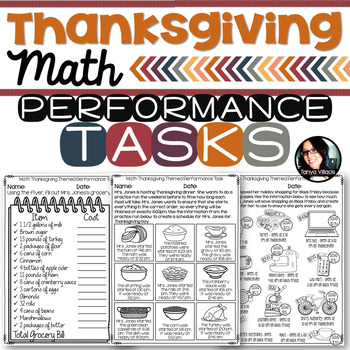# Thanksgiving Activities Math Worksheets Grades 4-64th - 6th, Homeschool
Subjects
Standards
Resource Type
Formats Included
• PDF
Pages
20 pages

### Description

Need to challenge your students? Want feedback on how your class is mastering the material you teach? Looking for more than a standard multiple choice assessment? Take your math instruction to the next level and get your students excited about math! PERFORMANCE TASKS ARE WHAT YOUR STUDENTS NEED.

This purchase contains 7 performance tasks aligned to the following math skills:

➥ 4.NF.1 - Equivalent Fractions

➥ 4.NF.3 - Adding Fractions with Like Denominators

➥ 4.MD.2 - Elapsed Time

➥ 4.MD.5 - Degrees in a Circle

➥ 4.MD.6 - Measuring using a Protractor

➥ 4.NBT.4 - Adding and Subtracting Whole Numbers

These performance tasks are meant to access conceptual understanding, along with procedural understanding.

Need more performance tasks for the classroom? Check out the resources below.

Area and Perimeter Performance Tasks COMMON CORE ALIGNED 4.MD.3

5 DIFFERENTIATED FRACTION PERFORMANCE TASKS **COMMON CORE ALIGNED** 4.NF.1

Total Pages
20 pages
Included
Teaching Duration
N/A
Report this Resource to TpT
Reported resources will be reviewed by our team. Report this resource to let us know if this resource violates TpT’s content guidelines.

### Standards

to see state-specific standards (only available in the US).
Explain why a fraction ð¢/ð£ is equivalent to a fraction (ð¯ Ã ð¢)/(ð¯ Ã ð£) by using visual fraction models, with attention to how the number and size of the parts differ even though the two fractions themselves are the same size. Use this principle to recognize and generate equivalent fractions.
Understand a fraction ð¢/ð£ with ð¢ > 1 as a sum of fractions 1/ð£.
Use the four operations to solve word problems involving distances, intervals of time, liquid volumes, masses of objects, and money, including problems involving simple fractions or decimals, and problems that require expressing measurements given in a larger unit in terms of a smaller unit. Represent measurement quantities using diagrams such as number line diagrams that feature a measurement scale.
Recognize angles as geometric shapes that are formed wherever two rays share a common endpoint, and understand concepts of angle measurement:
An angle is measured with reference to a circle with its center at the common endpoint of the rays, by considering the fraction of the circular arc between the points where the two rays intersect the circle. An angle that turns through 1/360 of a circle is called a âone-degree angle,â and can be used to measure angles.